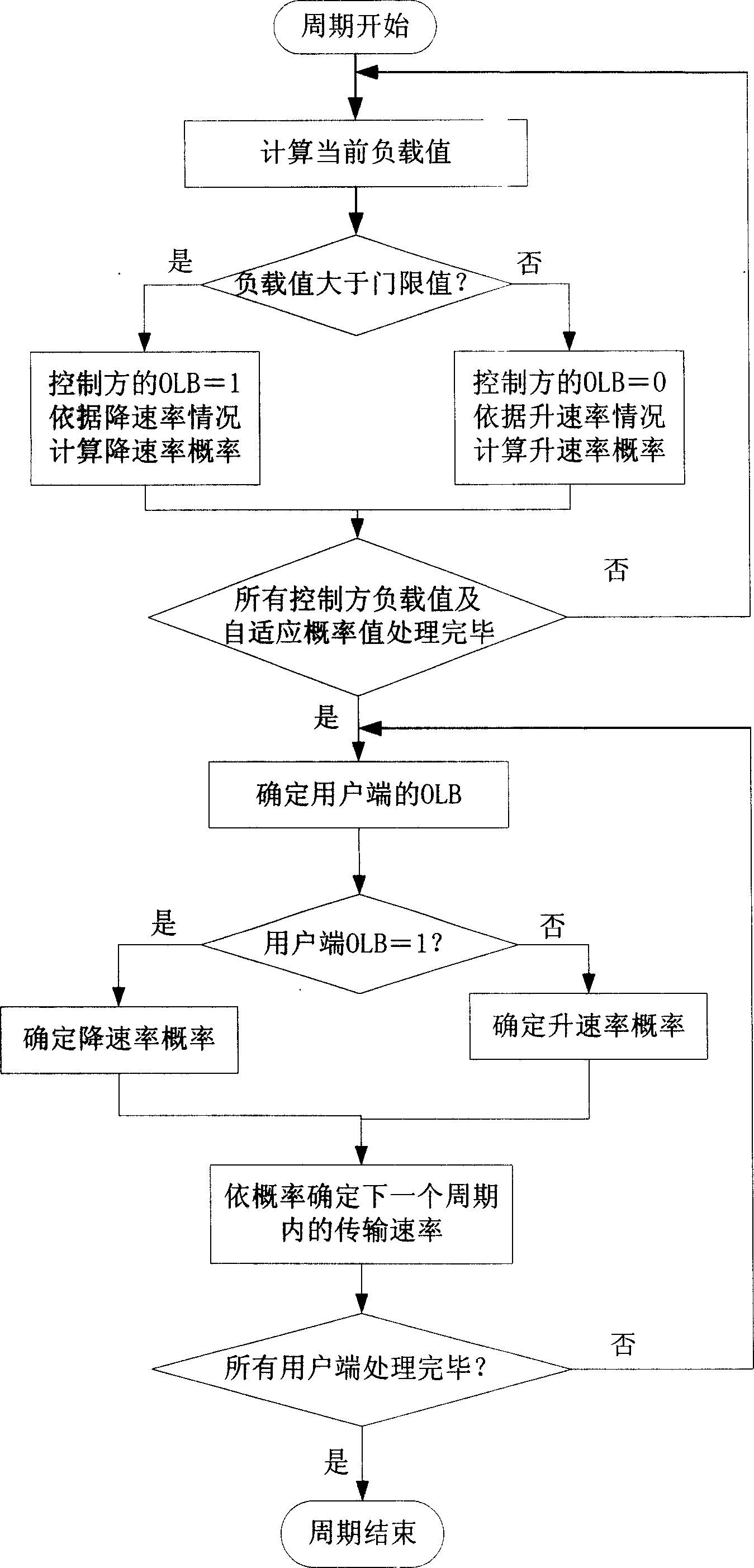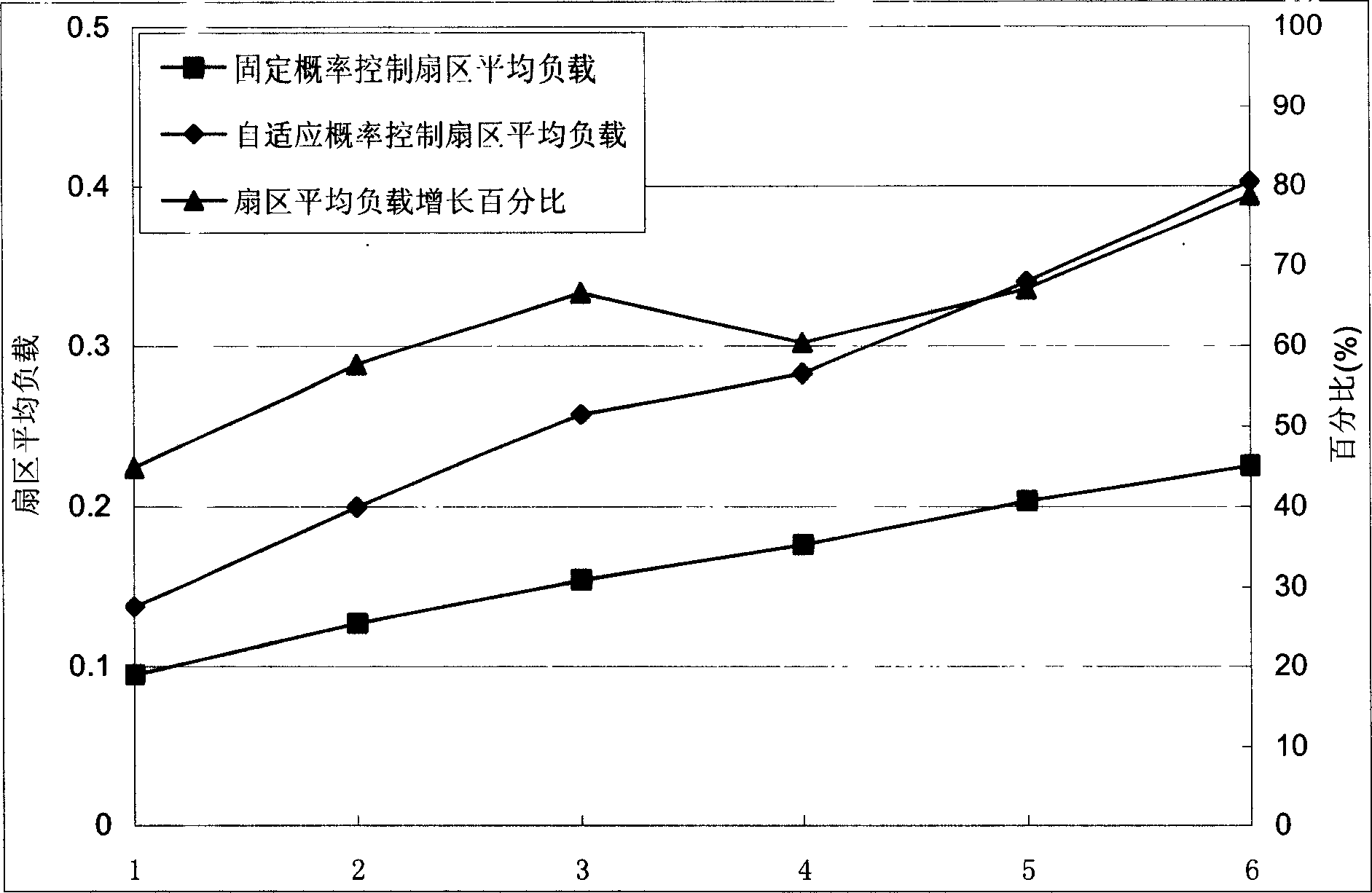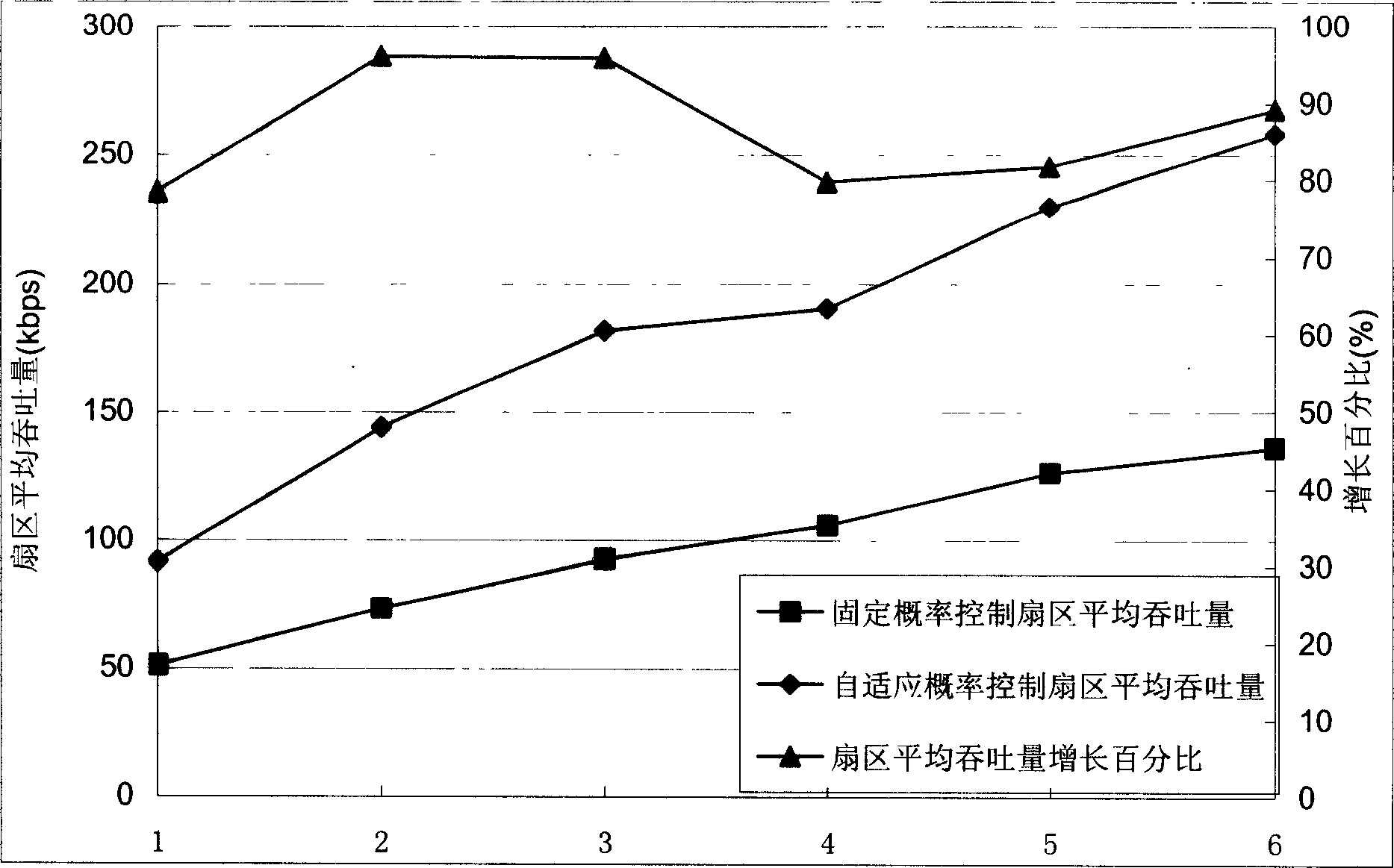## A rate control, mobile communication technology, applied in the direction of communication between multiple stations, can solve the problem of not being able to dynamically adapt to changes in system resource utilization

Inactive Publication Date: 2006-03-15
BEIJING UNIV OF POSTS & TELECOMM
0 Cites 19 Cited by

## AI-Extracted Technical Summary

### Problems solved by technology

The fixed probability adjustment method is easy to implement, but i...
View more

## Abstract

The invention can efficiently assign and dispatch resources in real time between many service requests to improve speed control method and utilization of system resources.

Application Domain

Technology Topic

## Image

•••## Examples

• Experimental program(1)

### Example Embodiment

 Because there are many implementation schemes for data services in mobile communication, in order to facilitate understanding, a representative and universally applicable example—CDMA2000 proposal (made by 3GPP2) is used here to illustrate the rate control method proposed by the present invention. The present invention is universally applicable to all applications with the basic features in this example.
 Use formula (1) to calculate the load contribution of user i to active set sector j:
 η i , j = 1 1 + W ( E b / N 0 ) i × R i × υ i - - - ( 1 )
 Among them, W is the system bandwidth, E b /N 0 Is the ratio of the user’s bit energy to the background thermal noise power spectral density, R i Is the transmission rate, υ i Is the voice activation factor. For data users, the voice activation factor is 1. According to the article "1xEV-DVEvaluation Methodology (V13)" of 3GPP2, the voice activation factor of the voice user is 0.406.
 The load value of a sector is equal to the sum of the load contributions of all users whose sector is the active set:
 Load j = Σ j ∈ ActiveSet ( i ) η i , j - - - ( 2 )
 The judgment method of the sector overload indication bit is:
 The article "IS-2000 Release C Simulation Configuration Specification" of 3GPP2 specifies the maximum load value, which is the load threshold Load TH Is 0.45. When the sector load is greater than 0.45, it indicates that the current sector is heavily loaded and the user should reduce the transmission rate; otherwise, it indicates that the current sector has remaining resources and the user can increase the transmission rate. However, the rate of increase and decrease of rate must be based on a certain probability, and not all users can increase or decrease the rate at the same time. If all users decrease the rate at the same time, the system resources will suddenly become idle. This idleness will cause all users to increase the rate during the next rate control cycle, resulting in system overload. Such reciprocation, the system fluctuates in idle and overload conditions, causing system instability. The main method of the present invention is to make the load value of the system as close as possible but not exceed the threshold value by adjusting the probability of rising and falling rates. The calculation method of the rate adjustment probability is detailed as follows.
 For the case where sector j allows users to increase the rate, that is, the current Load j TH , Suppose the current rate of user i is R i , The probability that the rate rises to twice the current rate is p j ↑ (0≤p j ↑ ≤1), keep the current rate R i The probability is l-p j ↑. Use rate estimation function Ev(R i , P j ↑ ) Calculate the rate of the next cycle and enter the load calculation formula. In this example, use the mathematical expectation function of the transmission rate in the next rate control period as the rate estimation function: Ev ( R i , p j ↑ ) = R ↑ ‾ = 2 × R i × p j ↑ + R i × ( 1 - p j ↑ ) = R i × ( 1 + p j ↑ ) . Will Bring in the load calculation formula to get
 Load j ‾ = Σ j ∈ ActiveSet ( i ) 1 1 + W ( E b / N o ) i × ( 1 + p j ↑ ) × R i × υ i - - - ( 4 )
 Formula (4) is variable p j ↑ (0≤p j ↑ ≤1) increasing function. Solve satisfies Load j ‾ Load TH Maximum probability under the condition p j ↑ , Which is the probability of the maximum rate of increase that makes sector j not overloaded.
 For the situation that sector j requires users to reduce the rate, that is, the current Liad j ≥Load TH , Suppose that user i reduces the rate to R i The probability of /2 is p j ↓ (0≤p j ↓ ≤1), keep the current rate R i Probability is 1-p j ↓. Also use the mathematical expectation function of the transmission rate in the next rate control period as the rate estimation function: Ev ( R i , p j ↓ ) = R ↓ ‾ = R i × p j ↓ / 2 + R i × ( 1 - p j ↓ ) = R i × ( 1 - p j ↓ / 2 ) . Each user Put it into the load calculation formula and get:
 Load j ‾ = Σ j ∈ ActiveSet ( i ) 1 1 + W ( E b / N o ) i × ( 1 - p j ↓ / 2 ) × R i × υ i - - - ( 5 )
 Formula (5) is variable p j ↓ (0≤p j ↓ ≤1) decreasing function. Solve satisfies Load j ‾ Load TH The smallest probability value p j ↓ As the rate reduction probability of changing sector j from overload to just over-loading, the full utilization of system resources is realized.
According to the "1xEV-DV Evaluation Methodology (V13)", the user's active set can contain up to three sectors. In order to meet the requirement that each activated sector is not overloaded, only when all activated sectors require a rate increase, the user will increase the rate of transmission, and the final rate rate increase probability is the minimum value of all rate increase probabilities. As long as there is an active set sector that requires the user to reduce the rate, the user will reduce the rate for transmission, and the rate of rate reduction shall be the maximum of all rate reduction probabilities. Therefore, the overload indicator bits finally determined by the user can be expressed as the sum of the overload indicator bits of the active set sector:
 OLB i = Σ j ∈ ActiveSet ( i ) OLB j - - - ( 6 )
 The probability selection method of user i is expressed as:
 p i ↑ = min j ∈ ActiveSet ( i ) p j ↑ RA B j = 0 , ( OLB i = 0 ) - - - ( 7 )
 p i ↓ = max j ∈ ActiveSet ( i ) RA B j = 1 p j ↓ , ( OLB i = 1 )
 Randomly generate a random number uniformly distributed in the range of [0, 1], if the random number is less than p i ↑ (p i ↑ ), the user will increase (decrease) the rate in the next rate control period to 2 times (1/2 times) the current rate.
 In addition, the present invention also takes into account the limitation of the available power of the system to the rate. Select the smaller rate among the maximum transmission rate that can be supported by the current available power and the transmission rate obtained by rate control as the final rate.
 Based on the above, it can be seen that the adaptive algorithm for real-time adjustment of rate rise and fall probability according to the system load condition proposed by the present invention has the following characteristics:
 1. For the purpose of improving the overall utilization efficiency of system resources, a greater system throughput is obtained for data services.
 2. Avoid the occurrence of system overload, while also ensuring the stability of the system.
 3. Considering high real-time performance, as the system conditions continue to change, adjust the rate rise and fall probability in real time to avoid blindness in the case of fixed probability.
 4. Insensitive to specific implementation methods and protocol specifications, and compatible.
 In short, the present invention is a flexible and practical resource allocation and scheduling technology, and its purpose is to improve the efficiency of resource allocation and rate adjustment in mobile communications with a simple structure and an easy-to-implement method.

## PUM## Description & Claims & Application Information

We can also present the details of the Description, Claims and Application information to help users get a comprehensive understanding of the technical details of the patent, such as background art, summary of invention, brief description of drawings, description of embodiments, and other original content. On the other hand, users can also determine the specific scope of protection of the technology through the list of claims; as well as understand the changes in the life cycle of the technology with the presentation of the patent timeline. Login to view more.
Who we serve
• R&D Engineer
• R&D Manager
• IP Professional
Why Eureka